# Triangles & Angles (10 Common Questions Answered)

Triangles come up often in mathematics, and for good reason – they are useful for a variety of purposes.  We can use their angles to categorize triangles and make statements about what they look like and how their sides are related.

A triangle’s angles sum to 180 degrees.  Triangles can have at most one right or obtuse angle.  A triangle has either two or three acute angles.  Isosceles triangles have two sides (and angles) that are the same.  Equilateral triangles have three sides (and angles) that are the same.

Of course, comparing the angles of triangles can also tell us about the sides.  For example, the largest angle of a triangle will be across from its longest side.

In this article, we’ll take a closer look at triangles and their angles.  We’ll also answer some common questions about triangles and what type of angles they can have.

Let’s get started.

## Triangles & Angles

A triangle is a polygon that always has exactly three sides and three angles.  Each of the sides is “across from” one of the angles.

The longest side will be across from the largest angle, and the smallest side will be across from the smallest angle.

No matter the shape of a triangle, its three angles will always add up to the same value.

## What Do The Angles In A Triangle Add Up To?

The angles in a triangle always add up to 180 degrees: no more, no less.

This article from Home School Math has a nice proof of this fact!

This restriction still leaves lots of possibilities for the angles in a triangle.  For example, we could have a triangle with:

• Angles of 10 degrees, 20 degrees, and 150 degrees (obtuse triangle)
• Angles of 30 degrees, 60 degrees, and 90 degrees (right triangle)
• Angles of 50 degrees, 60 degrees, and 70 degrees (acute triangle)
• A 40 degree angle and two 70 degree angles (isosceles triangle)
• A 90 degree angle and two 45 degree angles (right isosceles triangle)
• Three 60 degree angles (equilateral triangle)

There are many more possibilities.  As you can see from some of these examples, a triangle can have an angle greater than or equal to 90 degrees.

However, this still leaves the question of how many such angles the triangle can have.  To answer this question, we’ll start by looking at obtuse triangles.

## When Is A Triangle Obtuse?

A triangle is obtuse when it has an obtuse angle.  An obtuse angle is an angle with a measure of more than 90 degrees.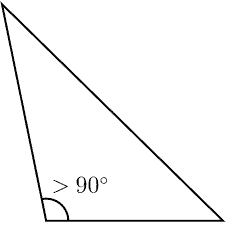An obtuse triangle has one obtuse angle (and only one) with a measure greater than 90 degrees.

An obtuse triangle is a type of oblique triangle (one that does not have a 90 degree angle).  An acute triangle is also oblique (more on acute triangles later).

Note that the other two angles in an obtuse triangle can be equal, but they do not have to be.

### Can A Triangle Have Two Obtuse Angles?

An obtuse triangle cannot have two (or three) obtuse angles.  An obtuse triangle has only one obtuse angle – the other two angles are acute angles, with measures less than 90 degrees.

The proof comes from the fact that the three angles in a triangle add up to 180 degrees.  If two angles in a triangle were obtuse (greater than 90 degrees), their sum would already be more than 180 degrees.

## When Is A Triangle A Right Triangle?

A triangle is a right triangle when it has a right angle.  A right angle is an angle with a measure of exactly 90 degrees.A right triangle has one right angle (and only one) with a measure of exactly 90 degrees.

This means that the two shortest sides (legs) of the right triangle are perpendicular to one another.  It also means that the other two angles (across from the legs) are complementary angles (they add up to 90 degrees).

For a right triangle, we can use the Pythagorean Theorem.  Remember that if the side lengths of a right triangle are a, b, and c (with c the hypotenuse, or the longest side, across from the 90 degree angle), then:

• a2 + b2 = c2

Without a 90 degree angle, we cannot use the Pythagorean Theorem (instead, we must use the Law of Cosines).

Note that the other two angles in a right triangle can be equal, but they do not have to be.

### Can A Triangle Have Two Right Angles?

A triangle cannot have two (or three) right angles.  An right triangle has only one right angle – the other two angles are acute angles, with measures less than 90 degrees (as mentioned earlier, their measures must add up to 90 degrees, since they are complementary angles).

The proof comes from the fact that the three angles in a triangle add up to 180 degrees.  If two angles in a triangle were right angles (equal to 90 degrees), their sum would already be 180 degrees.

This would mean that the third angle would be 0 degrees, which means the opposite side would have zero length, and we would not have a triangle at all.

## When Is A Triangle Acute?

A triangle is acute when it has three acute angles.  Another way of saying this is that the largest angle of the triangle is acute.An acute triangle has three acute angles, so each angle has a measure less than 90 degrees. There are no right or obtuse angles.

An acute angle is an angle with a measure of less than 90 degrees.

An acute triangle is a type of oblique triangle (one that does not have a 90 degree angle).

Note that two or even three of the angles in an acute triangle can be equal.

### Can A Triangle Have Two Acute Angles?

A triangle can have two acute angles – in fact, a triangle must have at least two acute angles.  An obtuse triangle and a right triangle have exactly two acute angles.

An acute triangle has exactly three acute angles.  The table below shows the number of acute, right, and obtuse angles for each type of triangle.

## When Is A Triangle Isosceles?

A triangle is isosceles when two of its angles are equal.  This also means that the two sides across from those angles have the same length.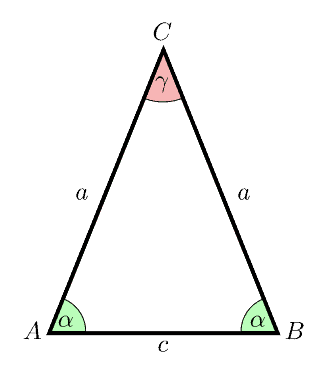An isosceles triangle has two equal angles (and the two sides across from those angles have the same length).

Let’s say that two of the angles in an isosceles triangle have the same measure of A.  Then the third angle has a measure of 180 – 2A.

Since no angle can have a negative measure, then we have:

• 180 – 2A >= 0
• 180 >= 2A
• 90 >= A

So, both of the angles that are equal in an isosceles triangle must also be acute angles.  There are three cases for the measure of the third angle, B:

• For 45 < A < 90 degrees: the third angle B is less than 90 degrees, and we have an acute isosceles triangle. For example, a triangle with angles 50 degrees, 50 degrees, and 80 degrees (80 degrees is the largest angle, but still less than 90 degrees, and so it is an acute angle).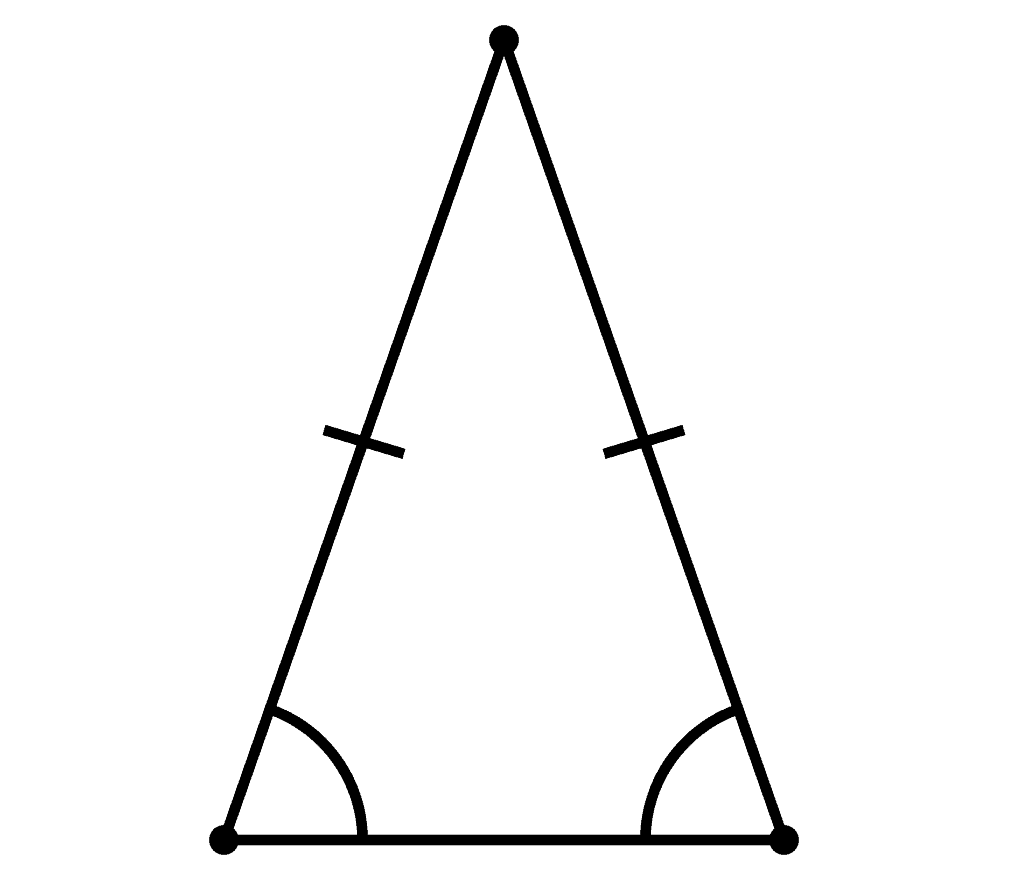An acute isosceles triangle has two equal acute angles (and thus two equal sides), and the third angle is also acute. So, all three angles are less than 90 degrees.
• For A = 45 degrees: the third angle B is 90 degrees, and we have a right isosceles triangle. For example, a triangle with angles 45 degrees, 45 degrees, and 90 degrees (90 degrees is the largest angle, so it is a right angle).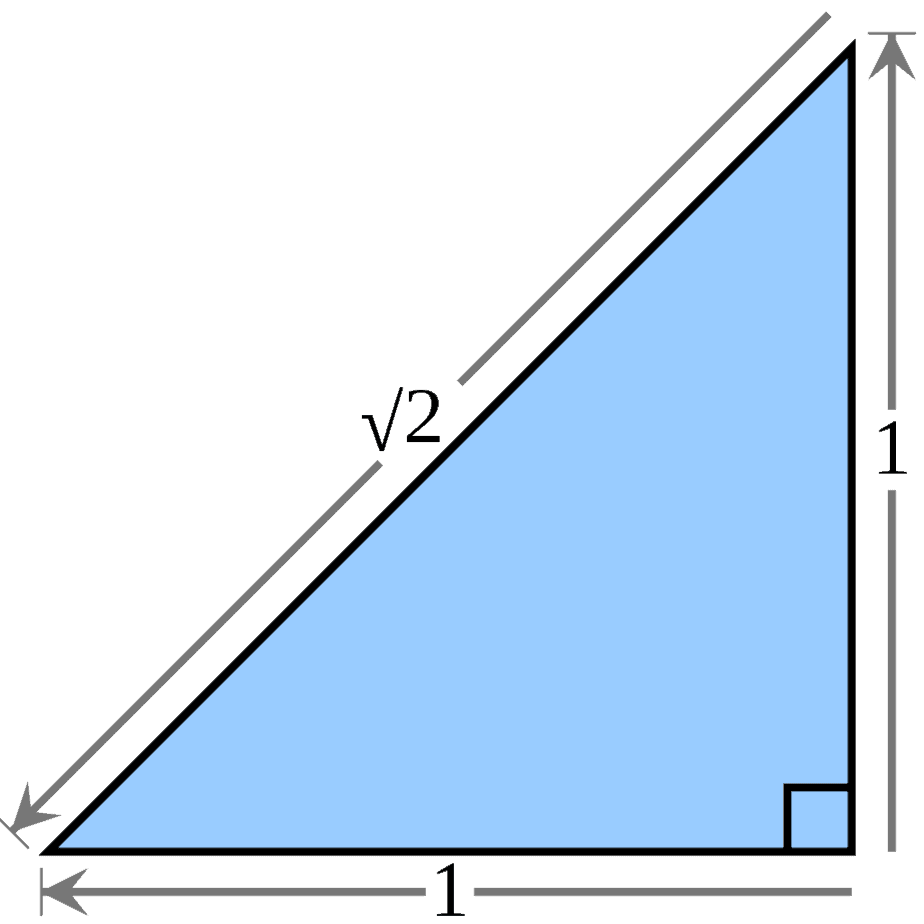A right isosceles triangle has two equal acute angles (and thus two equal sides), both of which have a measure of 45 degrees. The third angle is a right triangle, and it has a measure of 90 degrees.
• For A < 45 degrees: the third angle B is greater than 90 degrees, and we have an obtuse isosceles triangle. For example, a triangle with angles 40 degrees, 40 degrees, and 100 degrees (100 degrees is the largest angle, which is more than 90 degrees, and so it is an obtuse angle).

The table below summarizes this information for isosceles triangles.

If the third angle has the same measure as the other two angles (that is, A = 180 – 2A), then we have an equilateral triangle.

## When Is A Triangle Equilateral?

A triangle is equilateral when all three of its angles are equal.  This also means that the three sides across from those angles have the same length.

Let’s call A the measure of one of the angles in an equilateral triangle.  Then the three angles must add up to 180 degrees, so:

• A + A + A = 180
• 3A = 180
• A = 60

So, an equilateral triangle has three angles that each measure 60 degrees.  An equilateral triangle is a special case of an isosceles triangle, since three angles are the same (instead of just two being the same).

## Can A Triangle Have Parallel Sides?

A triangle cannot have parallel sides.  We can prove this by using the definition of parallel lines.

First, any pair of two sides (line segments) in a triangle will meet in a point at the vertex (one of the corners of the triangle).

However, two lines are parallel if they never meet.  So, two sides of a triangle cannot be parallel.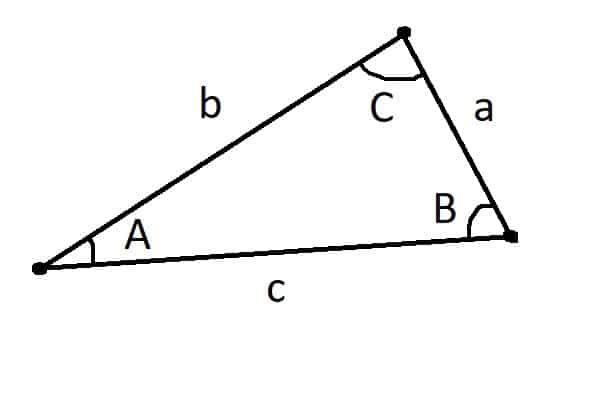Any two sides of a triangle will meet at a point (one of the vertices, or corners, of the triangle). Sides a and b meet at C, sides a and c meet at B, and sides b and c meet at A.

## Conclusion

Now you know more about triangles and their angles.  You also know the possibilities for acute, right, and obtuse angles within a triangle.

If you want to know more about lines and slopes, you can learn about when lines are parallel in my article here.

You can learn about the Triangle Inequality here.